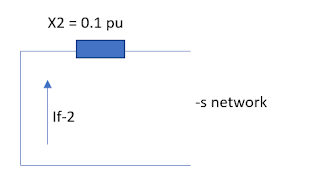The single line-to-ground fault on a transmission line happens when one conductor accidentally to the ground or comes in some cases in contact with the neutral conductor. For this reason, the protective devices of a power system needs to be accurately responsive to avoid severe damage in the system.

See Unbalanced Fault Analysis: Single Line to Ground Fault

Indeed, it is very important to know the procedure of single line to ground fault calculation.

Related articles:

• Double Line to Ground Fault
• Line to line fault
• Symmetrical three phase fault

Example:
Assumed that the generator below is solidly grounded and neglect the fault impedance. Determine the phase currents and phase voltage at faulted location.Generator

Solution:

Compute the base value:
Choose: Sb = 20 MVA and kVb = 13.8 kV
then,
Ea = 20 MVA/ 20 MVA = 1 (angle 0) per unit.
Ibase = 20 MVA / (1.73 x 13.8 kV)
Ibase = 0.837 kA

Develop positive sequence network:System Positive- Sequence Network Equivalent
where,
• Ea = the generator EMF, which has a value of 1 (angle 0) per unit.
• Xd" = the positive sequence impedance of transformer (in subtransient state)
• If-1 = positive sequence current.
Develop negative sequence network:System Negative- Sequence Network Equivalent
where,
• If-2 = negative sequence current
• X2 = negative sequence reactance

Develop zero sequence network:System Zero-Sequence Network Equivalent
where,
• If-0 = zero sequence current
• X2 = zero sequence reactance
By principle, the single line to ground fault will develop and equivalent network where all sequence networks are connected in series.

then, If-1 = 1 (angle 0)/ (Z0 + Z1 + Z2) = -j 2.22 pu or 2.22 (angle -90)
also it means that, If-2 = If-0 = - j 2.22 pu

Multiplying the base value

Ibase = 0.837 kA
then, If-1 = 2.22 (angle -90) * (0.837 kA) = 1.86 kA (angle -90) --> actual value
Since all sequence currents are equal, thus:
• If-1 = 1.86 kA (angle -90) --> + S
• If-2 = 1.86 kA (angle -90)  --> -S
• If-0 = 1.86 kA (angle -90) --> 0S
Applying the formula of sequence to phase values matrix we can get:
• Fault current at A = 5.58 kA (angle -90 degrees)
• Fault current at B = 0
• Fault current at C = 0
The zero values of phases B and C confirmed that no fault current flows from it during fault condition.

Computing Voltage at the faulted point,

Looking at this diagram, the voltage values of the un-faulted phases (phase B and C) are the only one that has a value while phase A (the faulted phase), its voltage is equal to zero (neglecting impedance).

Thus,

• Voltage at phase A = 0
• Voltage at phase B = Vb
• Voltage at phase C = Vc.
Analyzing positive sequence network equivalent,
• Vf-1 = 1 (angle 0) - (If-1) * (Z1) = 1 - (-j 2.22) (j 0.25) = 0.445 (angle 0) (+ sequence voltage)
Analyzing negative sequence network equivalent,
• Vf-2 = 0 - (If2) * (Z2) = 0- (-j2.22) * (j0.1) = 0.222 (angle 180) ( neg. sequence voltage).
Analyzing zero sequence network equivalent,
• Vf-0 = 0 - (If-0)* (Z0) = 0- (-j 2.22) * (j0.1) = 0.222 (angle 180) (zero sequence voltage).
Applying the formula of sequence to phase values matrix we can get:
• Voltage at phase A = 0
• Voltage at phase B = 0.667 (angle -120) pu
• Voltage at phase C = 0.667 (angle 120) pu
Applying base value,

Vbase = 13.8 kV/ 1.73

Therefore, the actual values of phase voltages are,
• Voltage at phase A = 0
• Voltage at phase B = 5.31 kV (angle -120)
• Voltage at phase C = 5.31 kV (angle 120)